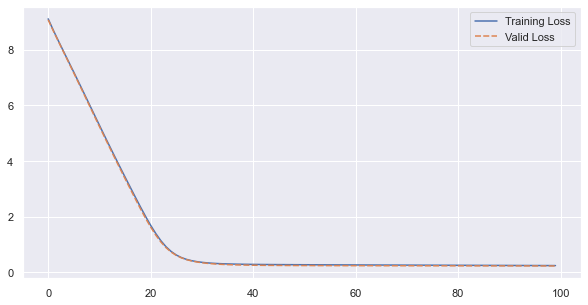# LSTM based auto-encoder returns flat line average

After a lot of struggling, I was able to implement a version of an autoencoder that uses an LSTM’s final hidden state as the encoding.

It trains with a pretty loss curve:but the decoder just outputs the average of the sequence (after a warm-up):I’m wondering what could be causing this kind of behavior in an autoencoder?

Encoder Class:

``````class SeqEncoderLSTM(nn.Module):
def __init__(self, n_features, latent_size):
super(SeqEncoderLSTM, self).__init__()

self.lstm = nn.LSTM(
n_features,
latent_size,
batch_first=True)

def forward(self, x):
_, hs = self.lstm(x)
return hs
``````

Decoder class:

``````class SeqDecoderLSTM(nn.Module):
def __init__(self, emb_size, n_features):
super(SeqDecoderLSTM, self).__init__()

self.cell = nn.LSTMCell(n_features, emb_size)
self.dense = nn.Linear(emb_size, n_features)

def forward(self, hs_0, seq_len):

# add each point to the sequence as it's reconstructed
x = torch.tensor([])

# Final hidden and cell state from encoder
hs_i, cs_i = hs_0

# reconstruct first (last) element
x_i = self.dense(hs_i)
x = torch.cat([x, x_i])

# reconstruct remaining elements
for i in range(1, seq_len):
hs_i, cs_i = self.cell(x_i, (hs_i, cs_i))
x_i = self.dense(hs_i)
x = torch.cat([x, x_i])

return x
``````

Bringing the two together:

``````
class LSTMEncoderDecoder(nn.Module):
def __init__(self, n_features, emb_size):
super(LSTMEncoderDecoder, self).__init__()
self.n_features = n_features
self.hidden_size = emb_size

self.encoder = SeqEncoderLSTM(n_features, emb_size)
self.decoder = SeqDecoderLSTM(emb_size, n_features)

def forward(self, x):
seq_len = x.shape
hs = self.encoder(x)
hs = tuple([h.squeeze(0) for h in hs])
out = self.decoder(hs, seq_len)
return out.unsqueeze(0)
``````

Since you’re getting the average, it means your network is underfitting the data. Either there aren’t patterns to exploit, or if there are, they’re not found, or at the very least not decoded by the LSTM.
I’d make sure:

• bottleneck dimension (LSTM’s hidden state) isn’t too small
• data has some patterns (i.e. not white noise)
• check that there’s variation in the hidden state, between data samples, by comparing with cosine distance. Use data samples with the same average as a control. If there’s very little variation it means the encoder is underfitting for sure. If there is variation for data with the same average, the decoder is failing somehow.

Thank you.

1. I’ve tried bottlenecks from 50 percent of original features to 150 percent. The issue persists.
2. The data is rather “unpredictable”. The paper I drew this architecture from advertises it as being excellent at reconstructing unpredictable sequences as compared to standard LSTM autoencoders. Perhaps they were too boastful?
3. I’ll try checking for variation. Thank you.

Also, my dataset is relatively small. I would think that overfitting would be the problem rather than under.

Hm, I’m relatively new to Pytorch so this is just a guess, but the line
`hs = tuple([h.squeeze(0) for h in hs])`

Looks like backprop probably stops here, as you’re leaving tensors for a tuple, which, unless there’s magic happening that isn’t obvious, doesn’t have a .grad_fn or .grad attribute… So how would the encoder get any gradients to train on?

Hmm! maybe! I’ve been eyeballing that line for two days wondering why I can’t find anyone else using something like that.

The `nn.LSTM` adds an undesired “batch_size” dimension that the `nn.LSTMCell` will reject. So I’ve got to squeeze it somehow… maybe in that process I’ve stopped backprop. But how else would I accomplish the squeeze?

Reshaping itself isn’t a problem for backprop, but a break in the chain of torch.Tensor / torch.Function will break the graph into fragments, and so I’m guessing only the decoder half is getting trained.

It’s hard to know what the shape of the tensors are from eyeballing the code, but I’m guessing some application of `hs.view(?, ?)` will do the trick.

I thought `squeeze` was a torch function? Anyway, I just retrained my using `view` instead with the same results.

My next thought is to use `nn.LSTMCell` in both the encoder and decoder. That way reshaping isn’t an issue since I don’t plan on using `batch_size > 1` anyway (If I can’t figure out this, I have no business making it more complicated).

Hi Wesley,just wondering if the issue has been solved. i experienced exactly the same issue when trying lstm and conv1d based architecture. thanks

Hey there, it’s been a while since I posted that but I think I solved it by passing the differences between point `i` and `i+1` instead of the raw data itself.